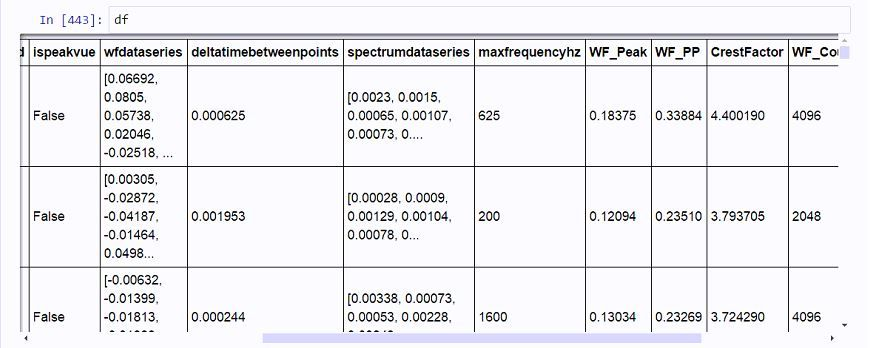# Support Questions

Announcements
Celebrating as our community reaches 100,000 members! Thank you!

## PySpark: How to add column to dataframe with calculation from nested array of floatsBrianWhite
New Contributor

Pardon, as I am still a novice with Spark. I am working with a Spark dataframe, with a column where each element contains a nested float array of variable lengths, typically 1024, 2048, or 4096. (These are vibration waveform signatures of different duration.)

An example element in the 'wfdataseries' colunmn would be [0.06692, 0.0805, 0.05738, 0.02046, -0.02518, ...]. A variety of metrics and statistics can be calculated from these blocks of vibration data.

The goal is to extract calculated features from each array, and place in a new column in the same dataframe. This is very easily accomplished with Pandas dataframes:

```from pyspark.sql import HiveContext, Row #Import Spark Hive SQLhiveCtx = HiveContext(sc) #Cosntruct SQL contextrows=hiveCtx.sql("SELECT collectiondate,serialno,system,accelerometerid,ispeakvue,wfdataseries,deltatimebetweenpoints,\
spectrumdataseries,maxfrequencyhz FROM test_vibration.vibrationblockdata")
import pandas as pd
df=rows.toPandas()
df['wfdataseries']=df.wfdataseries.apply(lambda x: x.encode('UTF8').split(','))
def str2flt(data): #Define function for converting list of strings to list of floats
return [float(value) for value in data]
df['wfdataseries']=df.wfdataseries.apply(str2flt)
df['WF_Peak']=df.wfdataseries.apply(lambda x: max(x)) #Calculate max value of nested array in each element of column 'wfdataseries'

# Various vibration waveform statistics
import numpy as np
from scipy import stats

df['WF_Var']=df.wfdataseries.apply(lambda x: np.var(np.array(x)))
df['WF_Kurt']=df.wfdataseries.apply(lambda x: stats.kurtosis(np.array(x),bias=True))
df['WF_Skew']=df.wfdataseries.apply(lambda x: stats.skew(np.array(x),bias=True))```Translating this functionality to the Spark dataframe has been much more difficult. The first step was to split the string CSV element into an array of floats. Got that figured out:

```from pyspark.sql import HiveContext #Import Spark Hive SQL
hiveCtx = HiveContext(sc) #Cosntruct SQL context
df=hiveCtx.sql("SELECT serialno,system,accelerometerid,ispeakvue,wfdataseries,deltatimebetweenpoints,\
spectrumdataseries,maxfrequencyhz FROM test_vibration.vibrationblockdata")
from pyspark.sql.functions import col, split
df = df.withColumn('wfdataseries',split(col('wfdataseries'),',').cast('array<float>'))```

But now, how do I use withColumn() to calculate the maximum of the nested float array, or perform any other calculation on that array? I keep getting "'Column' object is not callable".

Would an explode() method be needed in this case? I'd prefer something as elegant as Pandas if possible.

Also, I would need Numpy and Scipy calculations to be passed in for array calculations, as was easily done in Pandas.

```df = df.withColumn('WF_Peak',max('wfdataseries'))

---------------------------------------------------------------------------
TypeError                                 Traceback (most recent call last)
<ipython-input-97-be5735ba4c8a> in <module>()
----> 1 df = df.withColumn('WF_Peak',max('wfdataseries'))

TypeError: 'Column' object is not callable```
```df = df.withColumn('WF_Peak', df.wfdataseries.max())

---------------------------------------------------------------------------
TypeError                                 Traceback (most recent call last)
<ipython-input-99-a8b1116cac06> in <module>()
----> 1 df = df.withColumn('WF_Peak', df.wfdataseries.max())

TypeError: 'Column' object is not callable```

Suppose I stick with Pandas and convert back to a Spark DF before saving to Hive table, would I be risking memory issues if the DF is too large?

1 ACCEPTED SOLUTIONhubbarjaExpert Contributor

Hi Brian,

You shouldn't need to use exlode, that will create a new row for each value in the array.  The reason max isn't working for your dataframe is because it is trying to find the max for that column for every row in you dataframe and not just the max in the array.  Instead you will need to define a udf and call the udf within withColumn

```from pyspark.sql.functions import udf
def maxList(list):
max(list)
maxUdf==udf(scoreToCategory, FloatType())
df = df.withColumn('WF_Peak', maxUdf('wfdataseries'))```

As for using pandas and converting back to Spark DF, yes you will have a limitation on memory.  toPandas calls collect on the dataframe and brings the entire dataset into memory on the driver, so you will be moving data across network and holding locally in memory, so this should only be called if the DF is small enough to store locally.

3 REPLIES 3hubbarjaExpert Contributor

Hi Brian,

You shouldn't need to use exlode, that will create a new row for each value in the array.  The reason max isn't working for your dataframe is because it is trying to find the max for that column for every row in you dataframe and not just the max in the array.  Instead you will need to define a udf and call the udf within withColumn

```from pyspark.sql.functions import udf
def maxList(list):
max(list)
maxUdf==udf(scoreToCategory, FloatType())
df = df.withColumn('WF_Peak', maxUdf('wfdataseries'))```

As for using pandas and converting back to Spark DF, yes you will have a limitation on memory.  toPandas calls collect on the dataframe and brings the entire dataset into memory on the driver, so you will be moving data across network and holding locally in memory, so this should only be called if the DF is small enough to store locally.BrianWhite
New Contributor

Thanks, the UDF approach does work in this case.

```#Waveform peak amplitude
udf_wf_peak = udf(lambda x: max(x), returnType=FloatType()) #Define UDF function
df = df.withColumn('WF_Peak',udf_wf_peak('wfdataseries'))```

However, using Numpy arrays and functions has proven tricky, as the Numpy float dtype evidently does not match the Spark FloatType(). So in this case, where evaluating the variance of a Numpy array, I've found a work-around by applying round(x, 10), which converts it back. I suspect there's a more elegant solution, but that seems to work for now.

```#Waveform Variance
udf_wf_var = udf(lambda x: round(np.var(np.array(x)),10), returnType=FloatType()) #Define UDF function
df = df.withColumn('WF_Var',udf_wf_var('wfdataseries'))```hubbarjaExpert Contributor

Great, I'm glad the udf worked.  As for the numpy issue, I'm not familiar enough with using numpy within spark to give any insights, but the workaround seems trivial enough.  If you are looking for a more elegant solution, you may want to create a new thread and include the error.  You may also want to take a look at sparks mllib statistics functions, though they operate across rows instead of within a single column.